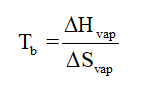# Chlorofluorocarbons (CFCs) are no longer used as refrigerants because they destroy the ozone layer.Trichlorofluoromethane (CCl3F) boils at 296.95 K, and its molar heat of vaporization is 24.8 kJ/mol.Calculate the molar entropy of evaporation of CCl3F(ℓ).

Question
2 views

Chlorofluorocarbons (CFCs) are no longer used as refrigerants because they destroy the ozone layer.
Trichlorofluoromethane (CCl3F) boils at 296.95 K, and its molar heat of vaporization is 24.8 kJ/mol.

Calculate the molar entropy of evaporation of CCl3F().

check_circle

Step 1

Boiling point, standard enthalpy of vaporization and standard entropy of vaporization are related by the equation given below:...

### Want to see the full answer?

See Solution

#### Want to see this answer and more?

Solutions are written by subject experts who are available 24/7. Questions are typically answered within 1 hour.*

See Solution
*Response times may vary by subject and question.
Tagged in